Next: Topological Quantum Field Theory Up: Planck Previous: Introduction

# 2. The Planck Length

Two constants appear throughout general relativity: the speed of light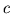and Newton's gravitational constant. This should be no surprise, since Einstein created general relativity to reconcile the success of Newton's theory of gravity, based on instantaneous action at a distance, with his new theory of special relativity, in which no influence travels faster than light. The constantalso appears in quantum field theory, but paired with a different partner: Planck's constant. The reason is that quantum field theory takes into account special relativity and quantum theory, in whichsets the scale at which the uncertainty principle becomes important.

It is reasonable to suspect that any theory reconciling general relativity and quantum theory will involve all three constants,, and. Planck noted that apart from numerical factors there is a unique way to use these constants to define units of length, time, and mass. For example, we can define the unit of length now called the Planck length' as follows:This is extremely small: aboutmeters. Physicists have long suspected that quantum gravity will become important for understanding physics at about this scale. The reason is very simple: any calculation that predicts a length using only the constants,andmust give the Planck length, possibly multiplied by an unimportant numerical factor like.

For example, quantum field theory says that associated to any mass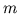there is a length called its Compton wavelength,, such that determining the position of a particle of massto within one Compton wavelength requires enough energy to create another particle of that mass. Particle creation is a quintessentially quantum-field-theoretic phenomenon. Thus we may say that the Compton wavelength sets the distance scale at which quantum field theory becomes crucial for understanding the behavior of a particle of a given mass. On the other hand, general relativity says that associated to any massthere is a length called the Schwarzschild radius,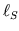, such that compressing an object of massto a size smaller than this results in the formation of a black hole. The Schwarzschild radius is roughly the distance scale at which general relativity becomes crucial for understanding the behavior of an object of a given mass. Now, ignoring some numerical factors, we have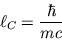and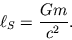These two lengths become equal whenis the Planck mass. And when this happens, they both equal the Planck length!

At least naively, we thus expect that both general relativity and quantum field theory would be needed to understand the behavior of an object whose mass is about the Planck mass and whose radius is about the Planck length. This not only explains some of the importance of the Planck scale, but also some of the difficulties in obtaining experimental evidence about physics at this scale. Most of our information about general relativity comes from observing heavy objects like planets and stars, for which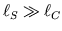. Most of our information about quantum field theory comes from observing light objects like electrons and protons, for which. The Planck mass is intermediate between these: about the mass of a largish cell. But the Planck length is abouttimes the radius of a proton! To study a situation where both general relativity and quantum field theory are important, we could try to compress a cell to a sizetimes that of a proton. We know no reason why this is impossible in principle. But we have no idea how to actually accomplish such a feat.

There are some well-known loopholes in the above argument. The unimportant numerical factor' I mentioned above might actually be very large, or very small. A theory of quantum gravity might make testable predictions of dimensionless quantities like the ratio of the muon and electron masses. For that matter, a theory of quantum gravity might involve physical constants other than,, and. The latter two alternatives are especially plausible if we study quantum gravity as part of a larger theory describing other forces and particles. However, even though we cannot prove that the Planck length is significant for quantum gravity, I think we can glean some wisdom from pondering the constantsand-- and more importantly, the physical insights that lead us to regard these constants as important.

What is the importance of the constant? In special relativity, what matters is the appearance of this constant in the Minkowski metricwhich defines the geometry of spacetime, and in particular the lightcone through each point. Stepping back from the specific formalism here, we can see several ideas at work. First, space and time form a unified whole which can be thought of geometrically. Second, the quantities whose values we seek to predict are localized. That is, we can measure them in small regions of spacetime (sometimes idealized as points). Physicists call such quantities local degrees of freedom'. And third, to predict the value of a quantity that can be measured in some region, we only need to use values of quantities measured in regions that stand in a certain geometrical relation to. This relation is called the causal structure' of spacetime. For example, in a relativistic field theory, to predict the value of the fields in some region, it suffices to use their values in any other region that intersects every timelike path passing through. The common way of summarizing this idea is to say that nothing travels faster than light. I prefer to say that a good theory of physics should have local degrees of freedom propagating causally.

In Newtonian gravity,is simply the strength of the gravitational field. It takes on a deeper significance in general relativity, where the gravitational field is described in terms of the curvature of the spacetime metric. Unlike in special relativity, where the Minkowski metric is a background structure' given a priori, in general relativity the metric is treated as a field which not only affects, but also is affected by, the other fields present. In other words, the geometry of spacetime becomes a local degree of freedom of the theory. Quantitatively, the interaction of the metric and other fields is described by Einstein's equation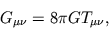where the Einstein tensor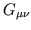depends on the curvature of the metric, while the stress-energy tensordescribes the flow of energy and momentum due to all the other fields. The role of the constantis thus simply to quantify how much the geometry of spacetime is affected by other fields. Over the years, people have realized that the great lesson of general relativity is that a good theory of physics should contain no geometrical structures that affect local degrees of freedom while remaining unaffected by them. Instead, all geometrical structures -- and in particular the causal structure -- should themselves be local degrees of freedom. For short, one says that the theory should be background-free.

The struggle to free ourselves from background structures began long before Einstein developed general relativity, and is still not complete. The conflict between Ptolemaic and Copernican cosmologies, the dispute between Newton and Leibniz concerning absolute and relative motion, and the modern arguments concerning the problem of time' in quantum gravity -- all are but chapters in the story of this struggle. I do not have room to sketch this story here, nor even to make more precise the all-important notion of geometrical structure'. I can only point the reader towards the literature, starting perhaps with the books by Barbour  and Earman , various papers by Rovelli [25,26,27], and the many references therein.

Finally, what of? In quantum theory, this appears most prominently in the commutation relation between the momentum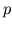and positionof a particle:together with similar commutation relations involving other pairs of measurable quantities. Because our ability to measure two quantities simultaneously with complete precision is limited by their failure to commute,quantifies our inability to simultaneously know everything one might choose to know about the world. But there is far more to quantum theory than the uncertainty principle. In practice,comes along with the whole formalism of complex Hilbert spaces and linear operators.

There is a widespread sense that the principles behind quantum theory are poorly understood compared to those of general relativity. This has led to many discussions about interpretational issues. However, I do not think that quantum theory will lose its mystery through such discussions. I believe the real challenge is to better understand why the mathematical formalism of quantum theory is precisely what it is. Research in quantum logic has done a wonderful job of understanding the field of candidates from which the particular formalism we use has been chosen. But what is so special about this particular choice? Why, for example, do we use complex Hilbert spaces rather than real or quaternionic ones? Is this decision made solely to fit the experimental data, or is there a deeper reason? Since questions like this do not yet have clear answers, I shall summarize the physical insight behindby saying simply that a good theory of the physical universe should be a quantum theory -- leaving open the possibility of eventually saying something more illuminating.

Having attempted to extract the ideas lying behind the constantsand, we are in a better position to understand the task of constructing a theory of quantum gravity. General relativity acknowledges the importance ofandbut idealizes reality by treatingas negligibly small. From our discussion above, we see that this is because general relativity is a background-free classical theory with local degrees of freedom propagating causally. On the other hand, quantum field theory as normally practiced acknowledgesandbut treatsas negligible, because it is a background-dependent quantum theory with local degrees of freedom propagating causally.

The most conservative approach to quantum gravity is to seek a theory that combines the best features of general relativity and quantum field theory. To do this, we must try to find a background-free quantum theory with local degrees of freedom propagating causally. While this approach may not succeed, it is definitely worth pursuing. Given the lack of experimental evidence that would point us towards fundamentally new principles, we should do our best to understand the full implications of the principles we already have!

From my description of the goal one can perhaps see some of the difficulties. Since quantum gravity should be background-free, the geometrical structures defining the causal structure of spacetime should themselves be local degrees of freedom propagating causally. This much is already true in general relativity. But because quantum gravity should be a quantum theory, these degrees of freedom should be treated quantum-mechanically. So at the very least, we should develop a quantum theory of some sort of geometrical structure that can define a causal structure on spacetime.

String theory has not gone far in this direction. This theory is usually formulated with the help of a metric on spacetime, which is treated as a background structure rather than a local degree of freedom like the rest. Most string theorists recognize that this is an unsatisfactory situation, and by now many are struggling towards a background-free formulation of the theory. However, in the words of two experts , `it seems that a still more radical departure from conventional ideas about space and time may be required in order to arrive at a truly background independent formulation.''

Loop quantum gravity has gone a long way towards developing a background-free quantum theory of the geometry of space [1,28], but not so far when it comes to spacetime. This has made it difficult to understand dynamics, and particular the causal propagation of degrees of freedom. Work in earnest on these issues has begun only recently. One reason for optimism is the recent success in understanding quantum gravity in 3 spacetime dimensions. But to explain this, I must first say a bit about topological quantum field theory.

Next: Topological Quantum Field Theory Up: Planck Previous: Introduction